Courses

# Olympiad Test: Multiplication - 1

## 20 Questions MCQ Test Mathematics Olympiad Class 4 | Olympiad Test: Multiplication - 1

Description
This mock test of Olympiad Test: Multiplication - 1 for Class 4 helps you for every Class 4 entrance exam. This contains 20 Multiple Choice Questions for Class 4 Olympiad Test: Multiplication - 1 (mcq) to study with solutions a complete question bank. The solved questions answers in this Olympiad Test: Multiplication - 1 quiz give you a good mix of easy questions and tough questions. Class 4 students definitely take this Olympiad Test: Multiplication - 1 exercise for a better result in the exam. You can find other Olympiad Test: Multiplication - 1 extra questions, long questions & short questions for Class 4 on EduRev as well by searching above.
QUESTION: 1

Solution:
QUESTION: 2

### Multiplication is the short form of repeated -------------

Solution:

Multiplication is the short from of repeated addition.

QUESTION: 3

### 43 × 0 =  ?

Solution:

We know 43 × 0 = 0

QUESTION: 4

66 × 1 = ?

Solution:
QUESTION: 5

74 × 21 = 21 × --------.

Solution:

We know 74 × 21 = 21 × 74 both are same

QUESTION: 6

543 × 10 = ?

Solution:
QUESTION: 7

23 × 100 = ?

Solution:

23 × 100 = 2300

QUESTION: 8

65 × -------- = 74 × 65

Solution:
QUESTION: 9

11 × 11 = ?

Solution:
QUESTION: 10

25 × 25 = ?

Solution:

Here 25 × 25 = 625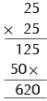QUESTION: 11

Pick the odd one out: 5, 10, 15, 20, 24

Solution:

Clearly 24 is not divisible by 5. All other are divisible.

QUESTION: 12

Pick the odd one out: 11, 22, 32, 44, 55

Solution:

Here 32 is odd one out.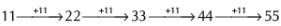∴ 32 = odd one out

QUESTION: 13

22 × 5 = ?

Solution:
QUESTION: 14

-------------- × 5 = 50

Solution:
QUESTION: 15

9 × 400 = ?

Solution:
QUESTION: 16

9 × 9 = ?

Solution:
QUESTION: 17

500 × 0 = ?

Solution:
QUESTION: 18

7899 × 1 = ?

Solution:
QUESTION: 19

500 × 400 = ?

Solution:

Here 500 × 400 = 20,0000
(∴ 5 × 4 = 20)

QUESTION: 20

------------ × 12 = 96

Solution:

Track your progress, build streaks, highlight & save important lessons and more!

### Similar Content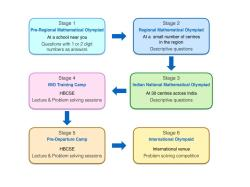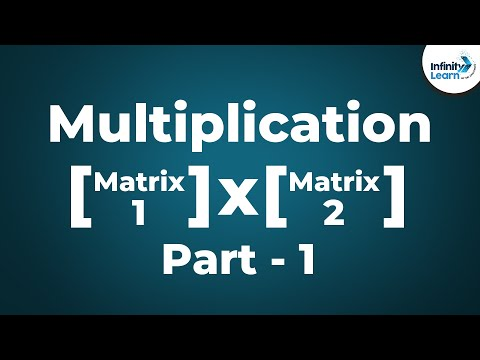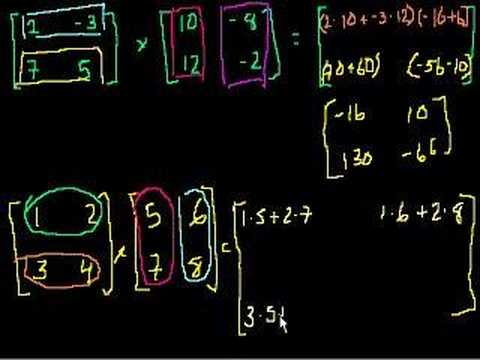### Related tests